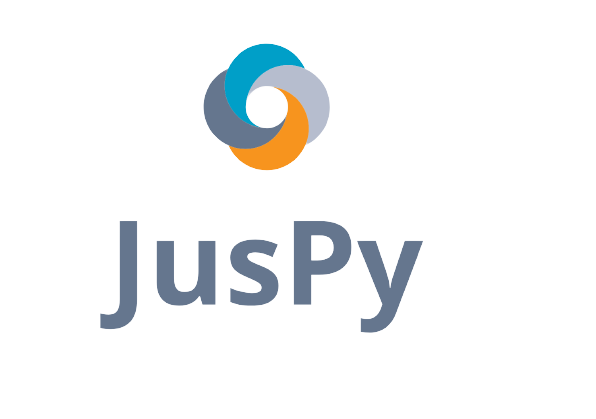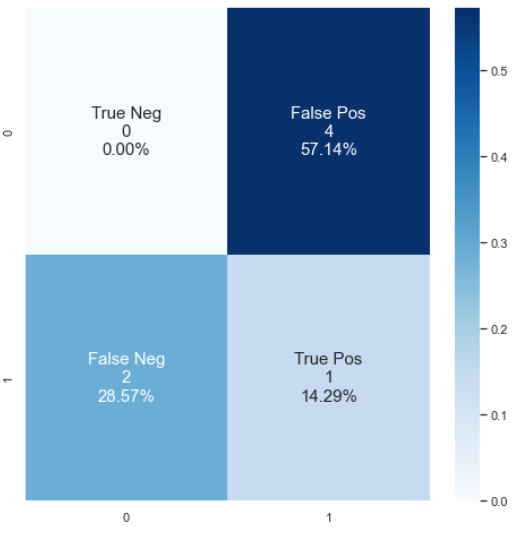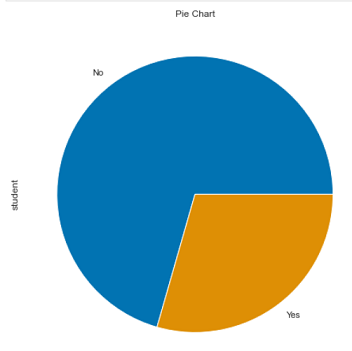An EDA and modellling assist library

# juspyjuspy is a library for making EDA and Modelling in Python quick and convinient. It's built on top of:

• pandas
• numpy
• seaborn
• and many such great libraries

Our reccomended path of getting started with juspy

• Open Anaconda Prompt
• In Anaconda Prompt type
conda install -c conda-forge jupyterlab

• and then
pip install juspy
jupyterlab


Incase if you're having any trouble regarding installation or dependencies, kindly make sure you're using updated versions

conda update conda
conda update pip
conda update python


And now try the above steps again

# Demo Notebooks:

1. To test library's proper installation and working:

import juspy
print(juspy.__version__)

from juspy import greet
print(greet.namastey())


Or, try greeting

print(greet.hello())
print(greet.ni_hao())
print(greet.hola())
print(greet.bonjour())
print(greet.schatz())
print(greet.ahlan())
print(greet.privet())


2. If they're producing some output, we're good to go
3. jpplot.confusion_matrix()

import numpy as np
import seaborn as sns
from sklearn.metrics import confusion_matrix

y_true = [0, 1, 0, 1, 0, 1, 0]
y_pred = [1, 1, 1, 0, 1, 0, 1]
cf_matrix = confusion_matrix(y_true, y_pred)

from juspy import plot as jpplot
jpplot.confusion_matrix(cf_matrix)4. jpplot.piechart()

import pandas as pd

from juspy import plot as jpplot
jpplot.piechart(df_name["student"])5. ## Future Release:

6. from juspy.linear_models import LinearRegression

from juspy.linear_models import LinearRegression as lin_reg


## Project details0.0.2b14 pre-release0.0.2b13 pre-release

This version0.0.2b12 pre-release0.0.2b11 pre-release0.0.2b1 pre-release0.0.1

Uploaded source
Uploaded py3